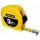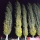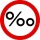Interior designer

To make draperies an interior designer needs 11 1/4 yards of material for the den and 8 1/2 yards for the living room. If material comes only in 20 yard bolts, how much will be left over after completing both sets of draperies?

Result

x =  0.25 yd

Solution:Leave us a comment of this math problem and its solution (i.e. if it is still somewhat unclear...):Be the first to comment!To solve this verbal math problem are needed these knowledge from mathematics:

Need help calculate sum, simplify or multiply fractions? Try our fraction calculator. Do you want to convert length units?

Next similar math problems:

1. Feet to milesA student runs 2640 feet. If the student runs an additional 7920 feet, how many total miles does the student run?
2. KingKing had four sons. First inherit 1/2, second 1/4 , third 1/5 of property. What part of the property was left to the last of the brothers?
3. Combine / add termCombine like terms 4c+c-7c
4. Conversion of unitsComplete the following length data
5. Length subtractingExpress in mm: 5 3/10 cm - 2/5 mm
6. Plan scaleAt what scale is drawn plan of the building, where one side of the building is 45 meters long is on the plan expressed by a straight line 12 mm long.
7. Turtles 2A box turtle hibernates in the sand at 11 5/8. A spotted turtle hibernates at 11 16/25 feet. Which turtle is deeper?
8. CupcakesIn a bowl was some cupcakes. Janka ate one third and Danka ate one quarter of cupcakes. a) How many of cookies ate together? b) How many cookies remain in a bowl? Write the results as a decimal number and in notepad also as a fraction.
9. Scale 3Miriam room is 3.2 meters wide. It is draw by line segment length 6.4 cm on floor plan. In what scale it is plan of the room?
10. SeamstressThe seamstress cut the fabric into 3 parts. The first part was the eighth fabric, the second part was three-fifths of the fabric and the third part had a length of 66 cm. Calculate the original length of the fabric.
11. PoplarHow tall is a poplar by the river, if we know that 1/5 of its total height is a trunk, 1/10th of the height is the root and 35m from the trunk to the top of the poplar?
12. UN 1If we add to an unknown number his quarter, we get 210. Identify unknown number.
13. Cleaning windowsCleaning company has to wash all the windows of the school. The first day washes one-sixth of the windows of the school, the next day three more windows than the first day and the remaining 18 windows washes on the third day. Calculate how many windows ha
14. Sum of fractionsWhat is the sum of 2/3+3/5?
15. FractionsThree-quarters of an unknown number are 4/5. What is 5/6 of this unknown number?
16. Equation with fractionsSolve equation: ? It is equation with fractions.
17. Two municipalitiesThe horizontal distance between municipalities is 39 km. Average sinking is 7 per mille. What is the difference in height between these municipalities?